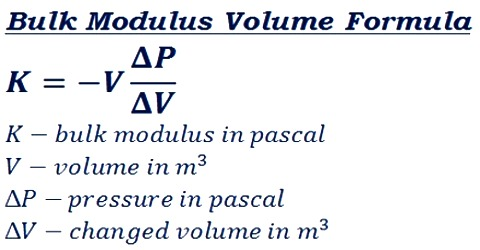Physics

# Bulk ModulusVolume or Bulk modulus: Within the elastic limit, the ratio of volume stress to volume strain is constant. This constant is called volume or bulk modulus. It is the ratio of the intensity of stress to the volume strain produced by stress —used of an elastic medium subjected to volume compression.

It is denoted by K.

Bulk/Volume modulus, K = [volume stress/volume strain]

If volume strain = 1, then K = volume stress.

So, within elastic limit the volume stress required to produce unit volume strain in a body is called volume or bulk modulus.Explanation: Let the initial volume of an object = V and the force applied normally on its unit area from all sides = p (force/area). If the change in volume is v, then volume strain = v/V and volume stress = p

so, K = [volume stress/volume strain] = P / (v/V) = PV/v

Whatever may be the pressure, the volume strain because very small for solids and liquids but for gases volume strain is quite large.

If the original volume of an object be V, the change in volume may be denoted by — ∆V, when the pressure increases by a small amount ∆p; the minus sign shows that the volume decreases.

Thus; K = – ∆P / (∆V/V) = V.∆p / ∆V

When the bulk modulus is constant (independent of pressure), this is a specific form of Hooke’s law of elasticity.